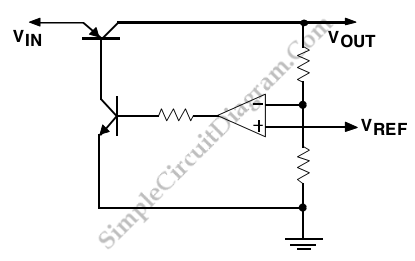# Low-Dropout (LDO) Voltage Regulator

This is a Low-dropout (LDO) Regulator circuit, constructed by using only a single PNP transistor. This circuit is directly related to load current. At very low values of load current as little as 50 mV. This circuit has maximum specified dropout voltage about 0.7V to 0.8V at full current and typical values around 0.6V. Compared with the other regulator types, this circuit has lowest (best) dropout voltage specification. Here is the basic schematic diagram of the circuit:To maintain regulation this circuit need minimum voltage across the LDO regulator given by this equation
VD(min)= VCE

This circuit is used in many application especially battery-powered applications because this circuit can operate with higher efficiency and maximize the utilization of the available input voltage. The ground pin of this circuit is the highest of the three types because it is approximately equal to the load current divided by the single PNP transistor’s gain.

For example LP2953 LDO regulator and The LM2940. The LM2940 has ground pin current specification of 45 mA (max) at full current, so it requires a PNP gain of 22 or higher and LP2953 has a ground pin current of 28 mA, so it need a PNP gain of 9 or higher. [Source: National Semiconductor Application Note]